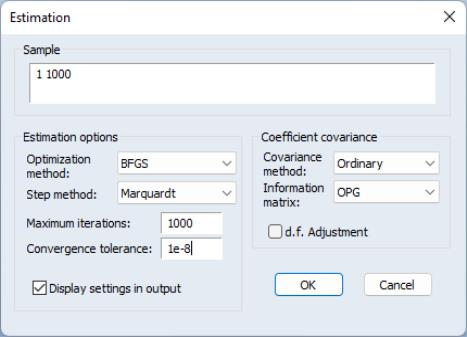Estimation
Once you have specified the logl object, you can ask EViews to find the parameter values which maximize the likelihood parameters. Simply click the Estimate button in the likelihood window toolbar to open the Estimation Options dialog.There are a number of options which allow you to control various aspects of the estimation procedure.
You may also use the Estimation options section of the dialog to specify the estimation method, step method, maximum number of iterations, convergence tolerance, and whether to display information about settings in the output.
Note that the default estimation method for new logl objects is BFGS.
The Coefficient covariance section of the dialog provides options for controlling the coefficient covariance estimate. The default Covariance method estimates the coefficient covariance using the inverse of the matrix specified in the Information matrix dropdown menu. Alternately, you may compute the sandwich covariance by selecting Huber – White in the Covariance method menu. By default, EViews uses the outer-product of the gradients (OPG) as the information matrix estimator, but if you are performing non-legacy optimization, you may substitute the (negative of the) observed Hessian by selecting Hessian – Observed.
See “Setting Estimation Options” for a discussion of these options.
The default settings should, however, provide a good start for most problems. When you click on OK, EViews will begin estimation using the current settings.
Starting Values
Since EViews uses an iterative algorithm to find the maximum likelihood estimates, the choice of starting values is important. For problems in which the likelihood function is globally concave, it will influence how many iterations are taken for estimation to converge. For problems where the likelihood function is not concave, it may determine which of several local maxima is found. In some cases, estimation will fail unless reasonable starting values are provided.
By default, EViews uses the values stored in the coefficient vector or vectors prior to estimation. If a @param statement is included in the specification, the values specified in the statement will be used instead.
In our conditional heteroskedasticity regression example, one choice for starting values for the coefficients of the mean equation coefficients are the simple OLS estimates, since OLS provides consistent point estimates even in the presence of (bounded) heteroskedasticity. To use the OLS estimates as starting values, first estimate the OLS equation by the command:
equation eq1.ls y c x z
After estimating this equation, the elements C(1), C(2), C(3) of the C coefficient vector will contain the OLS estimates. To set the variance scale parameter C(4) to the estimated OLS residual variance, you can type the assignment statement in the command window:
c(4) = eq1.@se^2
For the final heteroskedasticity parameter C(5), you can use the residuals from the original OLS regression to carry out a second OLS regression, and set the value of C(5) to the appropriate coefficient. Alternatively, you can arbitrarily set the parameter value using a simple assignment statement:
c(5) = 1
Now, if you estimate the logl specification immediately after carrying out the OLS estimation and subsequent commands, it will use the values that you have placed in the C vector as starting values.
As noted above, an alternative method of initializing the parameters to known values is to include a @param statement in the likelihood specification. For example, if you include the line:
@param c(1) 0.1 c(2) 0.1 c(3) 0.1 c(4) 1 c(5) 1
in the specification of the logl, EViews will always set the starting values to C(1)=C(2)=C(3)=0.1, C(4)=C(5)=1.